Processing ......FreeComputerBooks.com Links to Free Computer, Mathematics, Technical Books all over the World

Bayesian Methods for Hackers: Probabilistic Programming and Bayesian Inference
Top Free Computer Networking Books 🌠 - 100% Free or Open Source!
• Title: Bayesian Methods for Hackers: Probabilistic Programming and Bayesian Inference Using Python and PyMC
• Author(s) Cameron Davidson-Pilon
• Publisher: Addison-Wesley Professional (October 12, 2015); eBook (Online Edition. Updated Continuously)
• Paperback: 256 pages
• eBook: Jupyter Notebooks
• Language: English
• ISBN-10/ASIN: 0133902838
• ISBN-13: 978-0133902839Book Description

Master Bayesian Inference through Practical Examples and Computation - Without Advanced Mathematical Analysis.

Bayesian methods of inference are deeply natural and extremely powerful. However, most discussions of Bayesian inference rely on intensely complex mathematical analyses and artificial examples, making it inaccessible to anyone without a strong mathematical background. Now, though, Cameron Davidson-Pilon introduces Bayesian inference from a computational perspective, bridging theory to practice–freeing you to get results using computing power.

This book illuminates Bayesian inference through Probabilistic Programming with the powerful PyMC language and the closely related Python tools NumPy, SciPy, and Matplotlib. Using this approach, you can reach effective solutions in small increments, without extensive mathematical intervention.

This book is designed as an introduction to Bayesian inference from a computational understanding-first, and mathematics-second, point of view. The book assumes no prior knowledge of Bayesian inference nor probabilistic programming.

• Cameron Davidson-Pilon has seen many fields of applied mathematics, from evolutionary dynamics of genes and diseases to stochastic modeling of financial prices. His main contributions to the open-source community include Bayesian Methods for Hackers and lifelines. Cameron was raised in Guelph, Ontario, but was educated at the University of Waterloo and Independent University of Moscow. He currently lives in Ottawa, Ontario, working with the online commerce leader Shopify.
Reviews, Ratings, and Recommendations: Related Book Categories: Read and Download Links:Similar Books:
•Bayes Rules! An Introduction to Applied Bayesian Modeling

An engaging, sophisticated, and fun introduction to the field of Bayesian statistics, it brings the power of modern Bayesian thinking, modeling, and computing to a broad audience. Integrates R code, including RStan modeling tools, bayesrules package.

•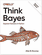O'Reilly® Think Bayes: Bayesian Statistics in Python

If you know how to program, you're ready to tackle Bayesian statistics. With this book, you'll learn how to solve statistical problems with Python code instead of mathematical formulas, using discrete probability distributions rather than continuous mathematics.

•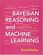Bayesian Reasoning and Machine Learning (David Barber)

This practical introduction is ideally suited to computer scientists without a background in calculus and linear algebra. You'll develop analytical and problem-solving skills that equip them for the real world. Numerous examples and exercises are provided.

•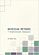Bayesian Methods for Statistical Analysis (Borek Puza)

Bayesian methods for statistical analysis is a book on statistical methods for analysing a wide variety of data. It contains many exercises, all with worked solutions, including complete computer code.

•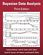Bayesian Data Analysis (Andrew Gelman, et al.)

This classic book is widely considered the leading text on Bayesian methods, lauded for its accessible, practical approach to analyzing data and solving research problems. It takes an applied approach to analysis using up-to-date Bayesian methods.

•An Introduction to Bayesian Thinking (Merlise Clyde, et al.)

This book provides an introduction to Bayesian inference in decision making without requiring calculus. It may be used on its own as an open-access introduction to Bayesian inference using R for anyone interested in learning about Bayesian statistics.

•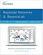Bayesian Networks and BayesiaLab (Stefan Conrady, et al.)

This practical introduction is geared towards scientists who wish to employ Bayesian Networks for applied research using the BayesiaLab software platform. It can serve as a self-study guide for learners and as a reference manual for advanced practitioners.

Book Categories
 :All CategoriesTop Free BooksRecent BooksMiscellaneous BooksComputer EngineeringComputer LanguagesComputer ScienceData Science/DatabasesJava and Java EE (J2EE)Linux and UnixMathematicsMicrosoft and .NETMobile ComputingNetworking and CommunicationsSoftware EngineeringSpecial TopicsWeb Programming
Other Categories